# GSEB Solutions Class 12 Statistics Part 2 Chapter 2 Random Variable and Discrete Probability Distribution Probability Ex 2.1

Gujarat Board Statistics Class 12 GSEB Solutions Part 2 Chapter 2 Random Variable and Discrete Probability Distribution Ex 2.1 Textbook Exercise Questions and Answers.

## Gujarat Board Textbook Solutions Class 12 Statistics Part 2 Chapter 2 Random Variable and Discrete Probability Distribution Ex 2.1

Question 1.
Examine whether the following distribution is a probability distribution of a discrete random variable X :
PM = $$\frac{x+2}{25}$$; x = 1, 2, 3, 4, 5
Here, p (x) = $$\frac{x+2}{25}$$
Putting, x = 1, 2, 3, 4, 5
P(1) = $$\frac{1+2}{25}$$ = $$\frac{3}{25}$$
P(2) = $$\frac{2+2}{25}$$ = $$\frac{4}{25}$$
P(3) = $$\frac{3+2}{25}$$ = $$\frac{5}{25}$$
P(4) = $$\frac{4+2}{25}$$ = $$\frac{6}{25}$$
P(5) = $$\frac{5+2}{25}$$ = $$\frac{7}{25}$$
Now, by the definition of discrete probability distribution, we must have
(1) p(x) > 0 and (2) Σp(x) = 1.
Now, p(xi) > 0 for (i = 1, 2, 3, 4, 5) and
Σp(xi) = p(1) + p(2) + p(3) + p(4) + p(5)
= $$\frac{3}{25}+\frac{4}{25}+\frac{5}{25}+\frac{6}{25}+\frac{7}{25}$$ = 1
Thus, conditions of probability distribution of discrete random variable are satisfied. So the given distribution is a probability distribution of a discrete random variable X.Question 2.
If the following distribution is a probability distribution of variable X, then find constant k.
P(x) = $$\frac{6-|x-7|}{k}$$; x = 4, 5, 6, 7, 8, 9, 10
Here, P(x) = $$\frac{6-|x-7|}{k}$$
Putting, x = 4, 5, 6, 7, 8, 9, 10
P(4) = $$\frac{6-|4-7|}{k}$$ = $$\frac{3}{k}$$
P(5) = $$\frac{6-|5-7|}{k}$$ = $$\frac{4}{k}$$
P(6) = $$\frac{6-|6-7|}{k}$$ = $$\frac{5}{k}$$
P(7) = $$\frac{6-|7-7|}{k}$$ = $$\frac{6}{k}$$
P(8) = $$\frac{6-|8-7|}{k}$$ = $$\frac{5}{k}$$
P(9) = $$\frac{6-|9-7|}{k}$$ = $$\frac{4}{k}$$
P(10) = $$\frac{6-|10-7|}{k}$$ = $$\frac{3}{k}$$
Now, by condition of probability distribution,
we must have
Σp(x) = 1
∴ P(4) + p(5) + P(6) + P(7) + P(8) + p(9) + P(10) = 1
∴ $$\frac{3}{k}+\frac{4}{k}+\frac{5}{k}+\frac{6}{k}+\frac{5}{k}+\frac{4}{k}+\frac{3}{k}$$
∴ $$\frac{30}{k}$$ = 1
∴ k = 30
Hence, the value of k obtained is 30.

Question 3.
The probability distribution of a random variable X is defined as follows: P(x) = $$\frac{k}{(x+1) !}$$; x = 1, 2, 3 and k = constant Hence, find (i) constant k (ii) P(1 < x < 4).
Here, P(x) = $$\frac{k}{(x+1) !}$$
Putting x = 1, 2, 3,
P(1) = $$\frac{k}{(1+1) !}$$ = $$\frac{k}{2 !}$$ = $$\frac{k}{2}$$
P(2) = $$\frac{k}{(2+1) !}$$ = $$\frac{k}{3 !}$$ = $$\frac{k}{6}$$
P(3) = $$\frac{k}{(3+1) !}$$ = $$\frac{k}{4 !}$$ = $$\frac{k}{24}$$

(i) Now, by condition of probability distribution.
we must have
p(x) = 1
∴ p(1) + p(2) + p(3) = 1
∴ $$\frac{k}{2}+\frac{k}{6}+\frac{k}{24}$$ = 1
∴ $$\frac{12 k+4 k+k}{24}$$ = 1
∴ $$\frac{17 k}{24}$$ = 1
∴ k = $$\frac{24}{17}$$(ii) Now, P(1 < x < 4) = P(2) + P(3)
= $$\frac{k}{6}+\frac{k}{24}$$ = $$\frac{4 k+k}{24}$$ = $$\frac{5 k}{24}$$
Putting, k = $$\frac{24}{17}$$
p(1 < x < 4) = $$\frac{5 \times 24}{24 \times 17}$$ = $$\frac{5}{17}$$
Hence, (i) k = $$\frac{24}{17}$$ (ii) P(1 < X < 4) = $$\frac{5}{17}$$

Question 4.
The probability distribution of a random variable X is as follows: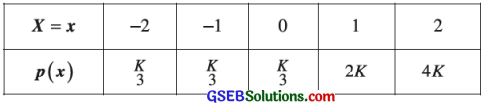Then (i) Determine acceptable value of constant k. (ii) Find the mean of the distribution.
(i) By condition of probability distribution, we must have
Σ p(x) = 1
∴ $$\frac{k}{3}+\frac{k}{3}+\frac{k}{3}$$ + 2k + 4k2 = 1
∴ $$\frac{k+k+k+6 k+12 k^{2}}{3}$$ = 1
∴ 9k + 12k2 = 3
∴ 12k2 + 9k – 3 = 0
∴ 3(4k2 + 3k – 1) = 0
∴ 4k2 + 4k – k – 1 = 0
∴ 4k (k+ 1) – 1(k + 1) = 0
∴ (4k- 1) (k + 1) = 0
∴ 4k – 1 = 0 or k + 1 = 0
∴ k = $$\frac{1}{4}$$ or k = – 1
If k = – 1, then p(- 2) = – $$\frac{1}{3}$$ which is not possible because p(x) > 0. So k = – 1 is not possible.
∴ Acceptable value is k = $$\frac{1}{4}$$.
Putting k = $$\frac{1}{4}$$, the probability distribution of random variable X is written as follows: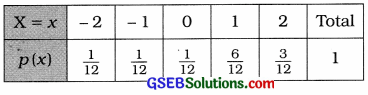(ii) Mean of the distribution:
µ = Σx ∙ p(x)
= – 2$$\left(\frac{1}{12}\right)$$ + – 1$$\left(\frac{1}{12}\right)$$ + 0$$\left(\frac{1}{12}\right)$$ + 1$$\left(\frac{6}{12}\right)$$ + 2$$\left(\frac{3}{12}\right)$$
= $$\frac{-2-1+0+6+6}{12}$$ = $$\frac{9}{12}$$ = $$\frac{3}{4}$$
Hence, the acceptable value of constant k is $$\frac{1}{4}$$ and the mean of the distribution obtained is $$\frac{3}{4}$$.Question 5.
The probability distribution of a random variable X is p (x). Variable X can assume the values x1 = – 2, x2 = – 1, x3 = 1 and x4 = 2 and if 4p(x1) = 2p (x2) = 3p (x3) = 4p (x4), then obtain mean and variance of this probability distribution.
p(x) is the probability distribution of a random variable X.
X = x1; x2, x3, x4 and x1 = – 2, x2 = – 1, x3 = 1, x4 = 2.
Σp(x) = 1
∴ p (x1) + p(x2) + p(x3) + p(x4) = 1 ……………… (1)
Now, 4p(x1) = 2p(x2) = 3p(x3) = 4p(x4)
∴ 4p (x1) = 4p(x4), 2p(x2) = 4p (x4) and 3p(x3) = 4p (x4)
∴ p(x1) = p(x4), p(x2) = 2p (x4) and
P(x3) = $$\frac{4 p\left(x_{4}\right)}{3}$$
Putting these values in the result (1),
p (x4) + 2p (x4) + $$\frac{4 p\left(x_{4}\right)}{3}$$ + p (x4) = 1
∴ $$\frac{3 p\left(x_{4}\right)+6 p\left(x_{4}\right)+4 p\left(x_{4}\right)+3 p\left(x_{4}\right)}{3}$$ = 1
∴ 16p (x4) = 3
∴ p (x4) = $$\frac{3}{16}$$
Now, P(x1) = P(x4) = $$\frac{3}{16}$$
P(x1) = 2P(x4)
= 2 × $$\frac{3}{16}$$
= $$\frac{6}{16}$$
P(x3) = $$\frac{4 p\left(x_{4}\right)}{3}$$
= $$\frac{4 \times 3}{3 \times 16}$$ = $$\frac{4}{16}$$
x1 = – 2, x2 = – 1, x3 = 1, and x4 = 2, the probability distribution of random variable X is written as follows:To find the mean and variance, the table is prepared as follows:Mean of the distribution:
µ = E (X) = Σx ∙ p(x) = –$$\frac{1}{8}$$

Variance of the distribution:
σ2 = V(X) = E (X2) – [E (X)]2
= Σx2 ∙ p(x) – [Σx – p(x)]2
= $$\frac{34}{16}-\left(-\frac{1}{8}\right)^{2}$$
= $$\frac{34}{16}-\frac{1}{64}$$
= $$\frac{136-1}{64}$$ = $$\frac{135}{64}$$
Hence, the mean and variance of the distribution obtained are $$\frac{1}{8}$$ and $$\frac{135}{64}$$ respectively.Question 6.
A die is randomly tossed two times. Determine the probability distribution of the sum of the numbers appearing both the times on the die and obtain expected value of the sum.
A die is randomly tossed two times.
∴ Total number of outcomes in the sample space U is n = 62 = 36.
For the sum of the numbers appearing both the times on the die is expressed as X = (u + v), where (u, v) is the element of U.

Now, the probability distribution X is obtained as shown in the following table: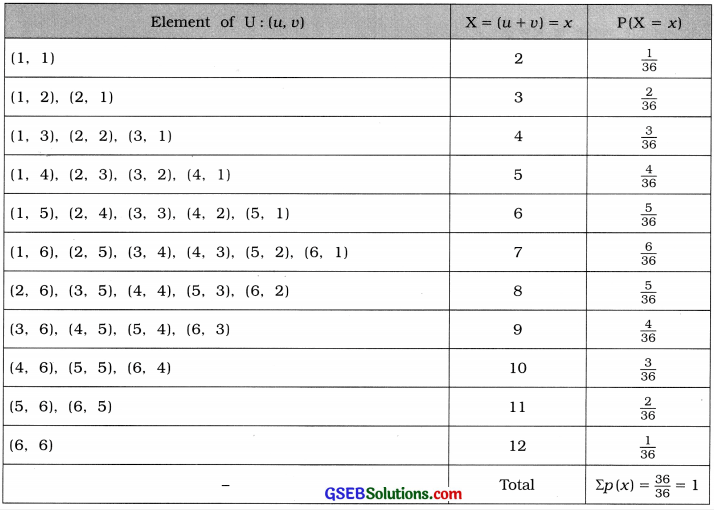The probability distribution of X is written as follows: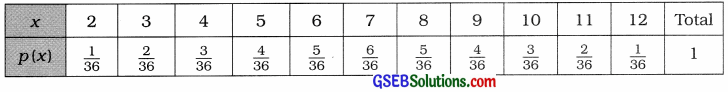Expected value of the sum:Hence, the expected value of the sum obtained is 7.

Alternative method: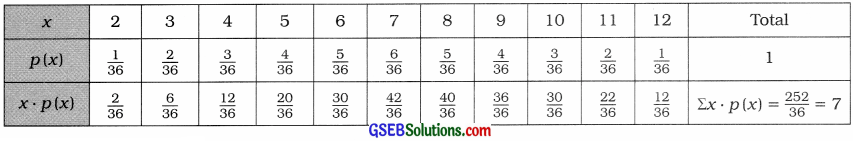Expected value of the sum:
E(X) = Σx ∙ p(x) = $$\frac{252}{36}$$ = 7Question 7.
A box contains 4 red and 2 blue balls. Three balls are simultaneously drawn at random. If X denotes the number of red bails in the selected balls, find the probability distribution of X and find the expected number of red balls in the selected balls.
In a box total (4 red + 2 blue) 6 balls.
3 balls are simultaneously drawn at random.
∴ Total number of primary outcomes n = 6C3 = $$\frac{6 \times 5 \times 4}{3 \times 2 \times 1}$$ = 20
X = No. of red balls in 3 balls drawn.
There are 4 red balls in a box.
∴ X = 0, 1, 2, 3.
The probability distribution of X is obtained as follows:Expected value of red balls:
E(X) = Σx ∙ p(x)
= 2
Hence, the expected value of red balls obtained is 2 balls.

Question 8.
A coin is tossed till either a head or 5 tails are obtained. If a random variable X denotes the necessary number of trials of tossing the coin, then obtain probability distribution of the random variable X and calculate its mean and variance.
A coin is tossed till either a head or 5 tails are obtained.
Suppose, X = Number of trials required for tossing a coin.
Here,
U = {(H), (TH), (TTH), (TTTH), (TTTTH), (TTTTT)}
∴ X = 1, 2, 3, 4, 5, 5

The following table shows the probability distribution of X: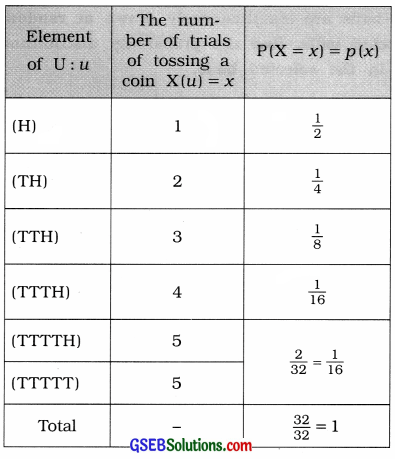To find the mean and variance of the distribution, the following table is prepared: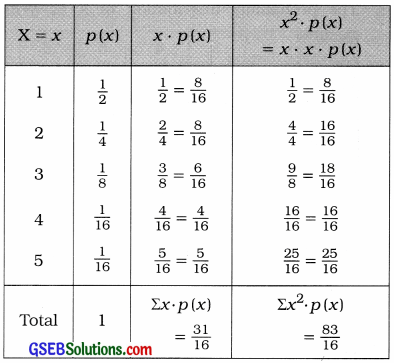Mean of the distribution:
µ = E(X)
= Σx ∙ p(x) = $$\frac{31}{16}$$
Variance of the distribution:
σ2 = V(X) = E(X2) – [E(X)]2
= Σx2 ∙ p(x) ∙ [Σx ∙ p(x)]2
= $$\frac{83}{16}-\left(\frac{31}{16}\right)^{2}$$
= $$\frac{83}{16}-\frac{961}{256}$$
= $$\frac{1328-961}{256}$$ = $$\frac{367}{256}$$
Hence, the mean and variance of the distribution obtained are $$\frac{31}{16}$$ and $$\frac{367}{256}$$ respectively.Question 9.
A shopkeeper has 6 tickets in a box. 2 tickets among them are worth a prize of ₹ 10 and the remaining tickets are worth a prize of ₹ 5. If a ticket is drawn at random from the box, find the expected value of the prize.
In a box there are total 6 tickets of which 2 tickets are with a prize of ₹ 10 and 4 tickets are with a prize of ₹ 5.
One ticket is drawn at random.
∴ Total number of primary outcomes n = 6C1 = 6
X = The value of prize
= 5, 10

The probability distribution of X is obtained as follows: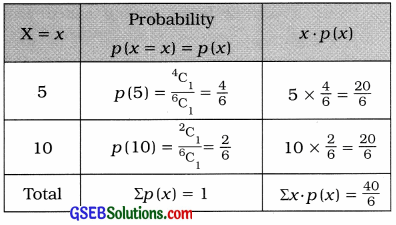The expected value of the prize:
E(X) = Σx ∙ P(x)
= $$\frac{40}{6}$$
= ₹ $$\frac{20}{3}$$
Hence, the expected value of the prize obtained is ₹ $$\frac{20}{3}$$